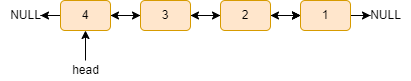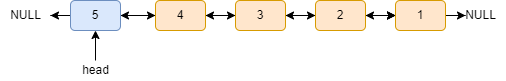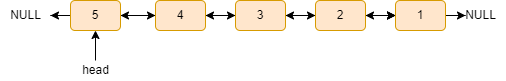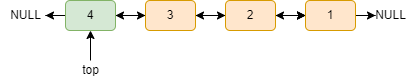# Implement stack using a doubly-linked listSandeep kamila
Last Updated: May 23, 2022
Difficulty Level :
MEDIUM

## Introduction

Stacks and Linked Lists are two of the most elementary Data Structures. They have applications across a wide range of problems and can prove to be very helpful. Here in this article, we are going to discuss how to implement a stack with the help of a doubly-linked list which should support the basic stack operations such as push(), pop(), top_element(), isEmpty(), and stack_size() following the Last in First Out model.

### Basic operations of stack

• push(): it pushes an element into the stack data structure.
• pop(): it removes the top element of the stack.
• top_element(): it gives the value of the top element of the stack.
• isEmpty(): it returns true if the stack is empty else returns false.
• print_stack(): it prints the contents of the stack in LIFO(last in first out) order.
• stack _size(): it gives the size of the stack.

## Algorithm

Doubly linked lists are a particular type of linked lists that can be traversed in both directions, i.e. forward (head to the last node) and backward direction (last node to head) (Check out Features of Doubly Linked List). The doubly linked list node has three fields: data, the next node’s address, and the previous node’s address.

The node of a Doubly linked list:To implement a stack using a doubly-linked list, we make some changes to make it work as a stack. Here the top of the stack is the head of the linked list. Initially, the head is NULL.

### Stack operations

#### push()

We attach the new node in front of the doubly linked list to follow LIFO(last in first out) order like the stack data structure.

Steps:

• Create a temp node of the given data.
• If the head is NULL, make the temp node the head node.
• Else make the next of the temp node as the head node, prev of the head node as the temp node, and prev of temp node as NULL.
• Finally, make the temp node the head node.

Example:

Suppose the doubly linked list looks like this:Now, after the push(5) operation, it will look like this:#### pop()

We remove the elements from the front of the linked list to follow the LIFO( last in first out) order as the stack data structure.

Steps:

• If the head is NULL, print “stack is empty”.
• If there is only one node in the list, create a temp node and store the head node, then make the head node NULL and delete the temp node.
• Else create a temp node and store the head in the temp node.
• Now, make head’s previous as NULL.
• Finally, delete the temp node to free the memory.

Example:

Suppose the list looks like this:After the pop() operation, it will look like this:#### top_element()

If the linked list is empty, print “stack is empty”. Else, print the data of the head node.

Example:

If the list looks like this:The top is:#### isEmpty()

If the head is NULL, return true else, return false.

#### stack_size()

Steps:

• Declare a count variable and initialize it with 0.
• Now, declare a curr pointer pointing to the head of the list.
• Run a while loop till curr!=NULL and do count++ and curr=curr->next.
• Finally, print the value of the count.

#### print_stack()

Steps:

• Declare a curr pointer pointing to the head of the list.
• Run a while loop till curr!=NULL.
• Print the curr->data and do curr=curr->next.

Before we get into the code, if you are familiar with the Singly Linked list, watch the video below to see how the stack is implemented using singly-linked lists; then, it will be a piece of cake for the Doubly Linked list.

### Code in C++

``````#include <bits/stdc++.h>
using namespace std;

struct Node {

struct Node* next;
int data;
struct Node* prev;
};

bool isEmpty() {
return true;

return false;
}
void top_element()
{
if (isEmpty())
cout << "stack is empty" << endl;
else
cout << "Top: " << head->data << endl;
}
void push(int data) {

struct Node* temp;
temp = new Node();
if (isEmpty())
{
temp->data = data;
temp->prev = NULL;
temp->next = NULL;
}
else
{   temp->data = data;
temp->prev = NULL;
}
}

void stack_size()
{
int count = 0;

while (curr != NULL)
{
count++;
curr = curr->next;
}

cout << "size: " << count << endl;
}

void pop() {

struct Node* temp;
temp = new Node();

if (isEmpty())
cout << "stack is empty" << endl;
{
}
else
delete(temp);
}
}

void print_stack() {

cout << "elements are: ";

while (curr != NULL)
{
cout << curr->data << " ";
curr = curr->next;

}

cout << endl;

}

int main() {

push(1);
push(2);
push(3);
push(4);
push(5);
print_stack();
stack_size();
top_element();
pop();
print_stack();
stack_size();
pop();
print_stack();
stack_size();
top_element();
return 0;
}``````

Output

``````Elements are: 5 4 3 2 1
Size: 5
Top: 5
Elements are: 4 3 2 1
Size: 4
Elements are: 3 2 1
Size: 3
Top: 3``````

### Complexity Analysis of different operations

Time complexity:

• push(): O(1) as we are not traversing the entire list.
• pop(): O(1) as we are not traversing the entire list.
• isEmpty(): O(1) as we are checking only the head node.
• top_element(): O(1) as we are printing the value of the head node only.
• stack_size(): As we traversed the whole list, it will be O(n), where n is the number of nodes in the linked list.
• print_stack(): As we traversed the whole list, it will be O(n), where n is the number of nodes in the linked list.

Space complexity: O(1), as we require constant extra space.

### What is a doubly-linked list used for?

It is utilized in navigation systems that require both forward and backward navigation. The browser operates a back and forward button to implement backward and forward navigation of visited web pages. It's also used to represent a standard deck of playing cards.

### What is the difference between stack and linked list?

A stack is an abstract data type representing a collection of components that may be manipulated using push and pop operations. On the other hand, a linked list is a linear collection of data components whose order is not determined by their memory location. This is the primary distinction between a stack and a linked list.

### How is stack used in memory management?

A stack is a memory section in a computer that keeps temporary variables generated by a function. Variables are declared, stored, and initialized in the stack during runtime. It's a type of transient memory. The memory of the variable will be automatically wiped once the computational process is completed.

## Conclusion

So, this article discussed the basics of a doubly-linked list, the implementation of stack using a doubly-linked list and the basic stack operations: push(), pop(), stack_sie(), isEmpty() and print_stack() with examples and its C++ code.

#### Recommended Problems

If you are a beginner, interested in coding, and want to learn Data Structures and Algorithms, you can look for our guided path for DSA on Codestudio which is free! Also, check out some Problems and Interview Experiences to gain an edge over the competition.